# Electrochemistry Class 12 NCERT Exercise Solutions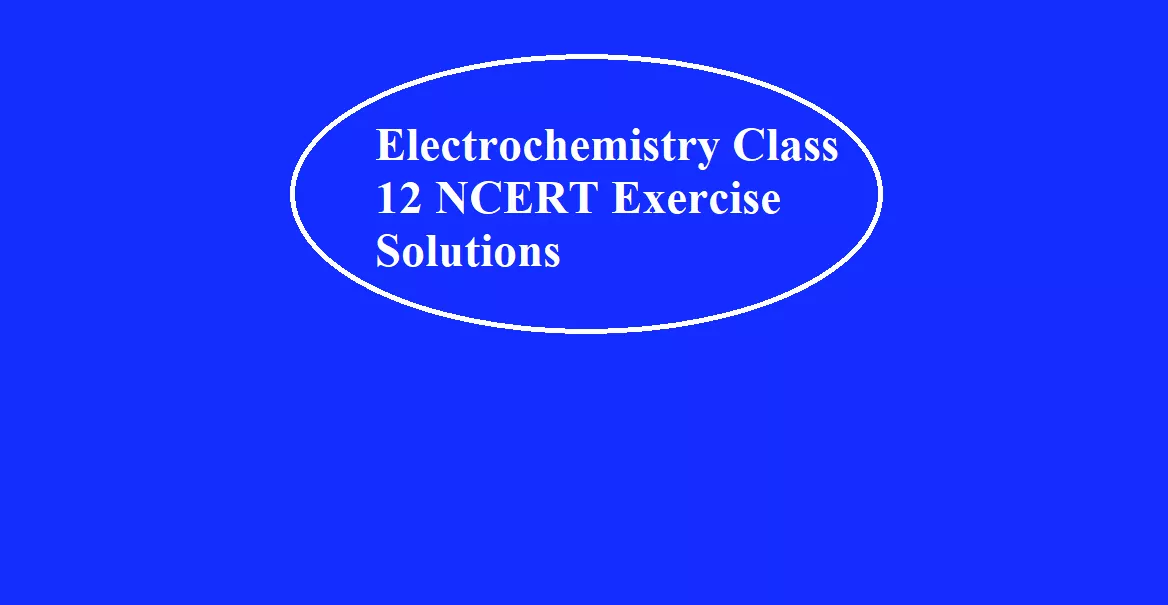Electrochemistry Class 12 NCERT Exercise Solutions

Que 1. Arrange the following metals in the order in which they displace each other from their salts. Al, Cu, Fe, Mg, and Zn
Ans 1. The order in which metals displace each other from the solution of their salts can be given with the help of their standard electrode potential. Since magnesium has the least standard electrode potential so it is the most strong reducing agent. So the required order we get is Mg, Al, Zn, Fe, Cu

Que 2. Given the standard electrode potentials, K+/K=-2. 93 V, Ag+/Ag = 0.80 V, Hg2+/Hg = 0.79V, Mg2+/Mg = -2.37V, Cr3+/Cr = 0.74V. Arrange these metals in their increasing order of reducing power.
Ans 2. Elements with reducing power or reducing agents have least/minimum standard electrode potential i.e., reducing power increases with a decrease in standard electrode potential. So the result obtained is K > Mg > Cr > Hg > Ag.

Que 3. Depict the galvanic cell in which the reaction
Zn(s) + 2Ag+(aq) 7M2+(aq) + 2Ag (s) takes place. Further show:
(i) Which of the electrode is negatively charged?
(ii) The carriers of the current in the cell.
(iii) Individual reaction at each electrode.
Ans 3. (i) The galvanic cell of the given reaction is depicted below :-
Zn (s) | Zn +2 (aq) || Ag (aq) | Ag (s)
Clearly Zn electrode is negatively charged.
(ii) The carriers of current in the cell are ions. So current flows from silver to zinc in the external circuit.
(iii)  At anode: Zn(s) → Zn2+(aq) + 2e
At cathode: 2Ag+(aq) + 2e → 2Ag(s)

Electrochemistry Class 12 NCERT Exercise Solutions

Que 4. Calculate the standard cell potentials of the galvanic cells in which the following reactions take place.
(a) 2Cr + 3Cd2+ → 2Cr3+ + 3Cd
Given E0 Cr3+/Cr = -0.74V, E0 Cd2+/Cd = -0.40V
(b) Fe2+ + Ag+ → Fe3+ + Ag
Given E0Ag+/Ag = 0.80V, E0Fe+3/Fe = 0.77V
Also, calculate ∆G° and equilibrium constant for the reaction.
Ans 4. Eø Cr3+ / Cr  = – 0.74 V
Eø Cd2+ / Cd  = – 0.40 V
The galvanic cell of the given reaction is depicted as:
Cr/Cr3+//Cd2+/Cd
Now, the standard cell potential is
Eø  = EøR  – EøL
= -0.40 – (-0.74) = + 0.34 V
ΔrGø = –nFEøcell
In the given equation,
n = 6
F = 96487 C mol – 1
Eøcell = +0.34 V
Then, ΔGø  = – 6 × 96487 C mol – 1 × 0.34 V
= – 196833.48 CV mol – 1
= – 196833.48 J mol – 1
= – 196.83 kJ mol – 1
Again,
ΔGø = – RT ln Kc
logKc = -ΔGø/2.303RT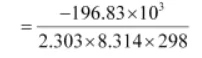= 34.496
K = antilog (34.496)
= 3.13 × 1034

(ii) Eø Fe3+ / Fe2+  =  0.77 V
Eø Ag+ / Ag        =  0.80 V
The galvanic cell of the given reaction is depicted as:
Fe+2/Fe3+//Ag+/Ag
Now, the standard cell potential is
Eø  = EøR  – EøL
= 0.80 – 0.77  = 0.03 V
Here, n = 1.
Then, ΔGø = –nFEøcell
= – 1 × 96487 C mol – 1 × 0.03 V
= – 2894.61 J mol – 1
= – 2.89 kJ mol – 1
Again, ΔGø = – 2.303 RT ln K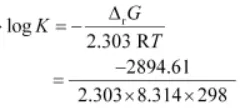= 0.5073
K = antilog (0.5073) = 3.2

Electrochemistry Class 12 NCERT Exercise Solutions

Que 5. Write the Nernst equation and emf of the following cells at 298 K:
(i) Mg(s) | Mg2+(0.001M) || Cu2+(0.0001 M) | Cu(s)
(ii) Fe(s) | Fe2+(0.001M) || H+(1M)|H2(g)(1bar) | Pt(s)
(iii) Sn(s) | Sn2+(0.050 M) || H+(0.020 M) | H2(g) (1 bar) | Pt(s)
(iv) Pt(s) | Br2(l) | Br(0.010 M) || H+(0.030 M) | H2(g) (1 bar) | Pt(s).
Ans 5. (i) Mg(s) +Cu2+(aq)↔ Mg2+(aq) + Cu(s)
Nernst equation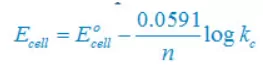There are two electron are transferring so that n = 2
cell = E°right – E°left
cell = + 0.34 – (–2.37)V
cell = +2.71V
Concentration of solid substance is 1 always so that [Mg] = [Cu] =1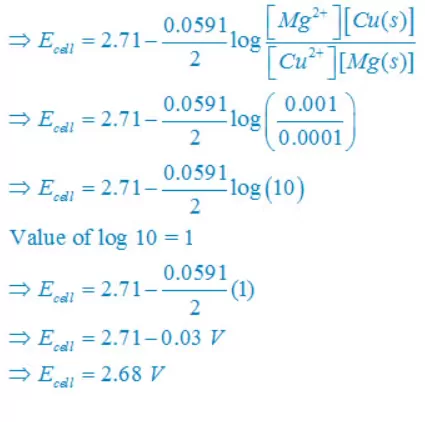(ii) Fe(s) +2H+(aq)↔ Fe2+(aq) + H2(g)
Nernst equationThere are two electrons are transferring so that n = 2
cell = E°right – E°left
cell =0– (–0.44)V
cell =+0.44 V
Concentration of solid substance is 1 always so that [Fe] = [H2] =1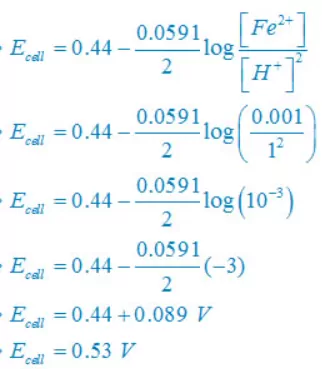(iii) Sn(s) +2H+(aq)↔ Sn2+(aq) + H2(g)
Nernst equationThere are two electrons are transferring so that n = 2
cell  = E°right – E°left
cell  = 0– (–0.14)V
cell  =+0.14 V
Concentration of solid substance is 1 always so that [Sn] = [H2] =1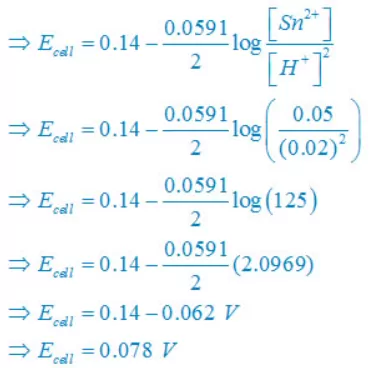(iv) 2Br(aq) + 2H+(aq)↔ Br2(l) + H2(g)
Nernst equationThere are two electron are transferring so that n = 2
cell  =  E°right – E°left
cell  = 0– (1.08)V

cell  = –1.08V
Concentration of solid substance is 1 always so that [Br2] = [H2] =1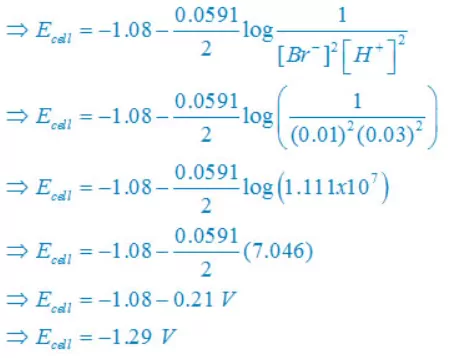Electrochemistry Class 12 NCERT Exercise Solutions